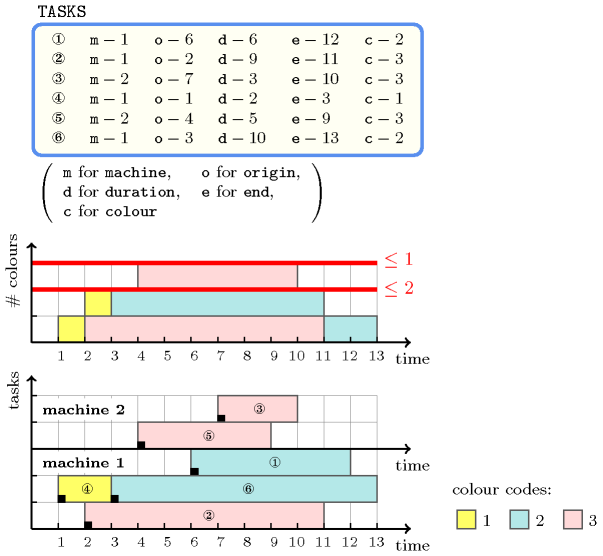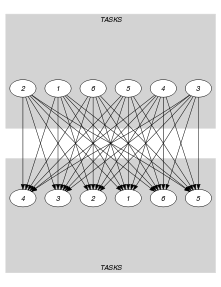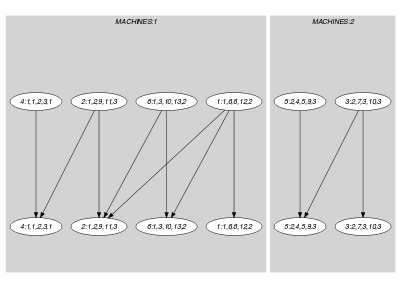## 5.74. coloured_cumulatives

Origin
Constraint

$\mathrm{𝚌𝚘𝚕𝚘𝚞𝚛𝚎𝚍}_\mathrm{𝚌𝚞𝚖𝚞𝚕𝚊𝚝𝚒𝚟𝚎𝚜}\left(\mathrm{𝚃𝙰𝚂𝙺𝚂},\mathrm{𝙼𝙰𝙲𝙷𝙸𝙽𝙴𝚂}\right)$

Synonym

$\mathrm{𝚌𝚘𝚕𝚘𝚛𝚎𝚍}_\mathrm{𝚌𝚞𝚖𝚞𝚕𝚊𝚝𝚒𝚟𝚎𝚜}$.

Arguments
 $\mathrm{𝚃𝙰𝚂𝙺𝚂}$ $\mathrm{𝚌𝚘𝚕𝚕𝚎𝚌𝚝𝚒𝚘𝚗}\left(\begin{array}{c}\mathrm{𝚖𝚊𝚌𝚑𝚒𝚗𝚎}-\mathrm{𝚍𝚟𝚊𝚛},\hfill \\ \mathrm{𝚘𝚛𝚒𝚐𝚒𝚗}-\mathrm{𝚍𝚟𝚊𝚛},\hfill \\ \mathrm{𝚍𝚞𝚛𝚊𝚝𝚒𝚘𝚗}-\mathrm{𝚍𝚟𝚊𝚛},\hfill \\ \mathrm{𝚎𝚗𝚍}-\mathrm{𝚍𝚟𝚊𝚛},\hfill \\ \mathrm{𝚌𝚘𝚕𝚘𝚞𝚛}-\mathrm{𝚍𝚟𝚊𝚛}\hfill \end{array}\right)$ $\mathrm{𝙼𝙰𝙲𝙷𝙸𝙽𝙴𝚂}$ $\mathrm{𝚌𝚘𝚕𝚕𝚎𝚌𝚝𝚒𝚘𝚗}\left(\mathrm{𝚒𝚍}-\mathrm{𝚒𝚗𝚝},\mathrm{𝚌𝚊𝚙𝚊𝚌𝚒𝚝𝚢}-\mathrm{𝚒𝚗𝚝}\right)$
Restrictions
 $\mathrm{𝚛𝚎𝚚𝚞𝚒𝚛𝚎𝚍}$$\left(\mathrm{𝚃𝙰𝚂𝙺𝚂},\left[\mathrm{𝚖𝚊𝚌𝚑𝚒𝚗𝚎},\mathrm{𝚌𝚘𝚕𝚘𝚞𝚛}\right]\right)$ $\mathrm{𝚛𝚎𝚚𝚞𝚒𝚛𝚎}_\mathrm{𝚊𝚝}_\mathrm{𝚕𝚎𝚊𝚜𝚝}$$\left(2,\mathrm{𝚃𝙰𝚂𝙺𝚂},\left[\mathrm{𝚘𝚛𝚒𝚐𝚒𝚗},\mathrm{𝚍𝚞𝚛𝚊𝚝𝚒𝚘𝚗},\mathrm{𝚎𝚗𝚍}\right]\right)$ $\mathrm{𝚃𝙰𝚂𝙺𝚂}.\mathrm{𝚍𝚞𝚛𝚊𝚝𝚒𝚘𝚗}\ge 0$ $\mathrm{𝚃𝙰𝚂𝙺𝚂}.\mathrm{𝚘𝚛𝚒𝚐𝚒𝚗}\le \mathrm{𝚃𝙰𝚂𝙺𝚂}.\mathrm{𝚎𝚗𝚍}$ $\mathrm{𝚛𝚎𝚚𝚞𝚒𝚛𝚎𝚍}$$\left(\mathrm{𝙼𝙰𝙲𝙷𝙸𝙽𝙴𝚂},\left[\mathrm{𝚒𝚍},\mathrm{𝚌𝚊𝚙𝚊𝚌𝚒𝚝𝚢}\right]\right)$ $\mathrm{𝚍𝚒𝚜𝚝𝚒𝚗𝚌𝚝}$$\left(\mathrm{𝙼𝙰𝙲𝙷𝙸𝙽𝙴𝚂},\mathrm{𝚒𝚍}\right)$ $\mathrm{𝙼𝙰𝙲𝙷𝙸𝙽𝙴𝚂}.\mathrm{𝚌𝚊𝚙𝚊𝚌𝚒𝚝𝚢}\ge 0$
Purpose

Consider a set $𝒯$ of tasks described by the $\mathrm{𝚃𝙰𝚂𝙺𝚂}$ collection. The $\mathrm{𝚌𝚘𝚕𝚘𝚞𝚛𝚎𝚍}_\mathrm{𝚌𝚞𝚖𝚞𝚕𝚊𝚝𝚒𝚟𝚎𝚜}$ constraint forces for each machine $m$ of the $\mathrm{𝙼𝙰𝙲𝙷𝙸𝙽𝙴𝚂}$ collection the following condition: at each point in time $p$, the numbers of distinct colours of the set of tasks that both overlap that point $p$ and are assigned to machine $m$ does not exceed the capacity of machine $m$. A task overlaps a point $i$ if and only if (1) its origin is less than or equal to $i$, and (2) its end is strictly greater than $i$. It also imposes for each task of $𝒯$ the constraint $\mathrm{𝚘𝚛𝚒𝚐𝚒𝚗}+\mathrm{𝚍𝚞𝚛𝚊𝚝𝚒𝚘𝚗}=\mathrm{𝚎𝚗𝚍}$.

Example
$\left(\begin{array}{c}〈\begin{array}{ccccc}\mathrm{𝚖𝚊𝚌𝚑𝚒𝚗𝚎}-1\hfill & \mathrm{𝚘𝚛𝚒𝚐𝚒𝚗}-6\hfill & \mathrm{𝚍𝚞𝚛𝚊𝚝𝚒𝚘𝚗}-6\hfill & \mathrm{𝚎𝚗𝚍}-12\hfill & \mathrm{𝚌𝚘𝚕𝚘𝚞𝚛}-2,\hfill \\ \mathrm{𝚖𝚊𝚌𝚑𝚒𝚗𝚎}-1\hfill & \mathrm{𝚘𝚛𝚒𝚐𝚒𝚗}-2\hfill & \mathrm{𝚍𝚞𝚛𝚊𝚝𝚒𝚘𝚗}-9\hfill & \mathrm{𝚎𝚗𝚍}-11\hfill & \mathrm{𝚌𝚘𝚕𝚘𝚞𝚛}-3,\hfill \\ \mathrm{𝚖𝚊𝚌𝚑𝚒𝚗𝚎}-2\hfill & \mathrm{𝚘𝚛𝚒𝚐𝚒𝚗}-7\hfill & \mathrm{𝚍𝚞𝚛𝚊𝚝𝚒𝚘𝚗}-3\hfill & \mathrm{𝚎𝚗𝚍}-10\hfill & \mathrm{𝚌𝚘𝚕𝚘𝚞𝚛}-3,\hfill \\ \mathrm{𝚖𝚊𝚌𝚑𝚒𝚗𝚎}-1\hfill & \mathrm{𝚘𝚛𝚒𝚐𝚒𝚗}-1\hfill & \mathrm{𝚍𝚞𝚛𝚊𝚝𝚒𝚘𝚗}-2\hfill & \mathrm{𝚎𝚗𝚍}-3\hfill & \mathrm{𝚌𝚘𝚕𝚘𝚞𝚛}-1,\hfill \\ \mathrm{𝚖𝚊𝚌𝚑𝚒𝚗𝚎}-2\hfill & \mathrm{𝚘𝚛𝚒𝚐𝚒𝚗}-4\hfill & \mathrm{𝚍𝚞𝚛𝚊𝚝𝚒𝚘𝚗}-5\hfill & \mathrm{𝚎𝚗𝚍}-9\hfill & \mathrm{𝚌𝚘𝚕𝚘𝚞𝚛}-3,\hfill \\ \mathrm{𝚖𝚊𝚌𝚑𝚒𝚗𝚎}-1\hfill & \mathrm{𝚘𝚛𝚒𝚐𝚒𝚗}-3\hfill & \mathrm{𝚍𝚞𝚛𝚊𝚝𝚒𝚘𝚗}-10\hfill & \mathrm{𝚎𝚗𝚍}-13\hfill & \mathrm{𝚌𝚘𝚕𝚘𝚞𝚛}-2\hfill \end{array}〉,\hfill \\ 〈\mathrm{𝚒𝚍}-1\mathrm{𝚌𝚊𝚙𝚊𝚌𝚒𝚝𝚢}-2,\mathrm{𝚒𝚍}-2\mathrm{𝚌𝚊𝚙𝚊𝚌𝚒𝚝𝚢}-1〉\hfill \end{array}\right)$

Figure 5.74.1 shows the solution associated with the example. Each rectangle of the figure corresponds to a task of the $\mathrm{𝚌𝚘𝚕𝚘𝚞𝚛𝚎𝚍}_\mathrm{𝚌𝚞𝚖𝚞𝚕𝚊𝚝𝚒𝚟𝚎𝚜}$ constraint. Tasks that have their colour attribute set to 1 and 2 are respectively coloured in blue and pink. The $\mathrm{𝚌𝚘𝚕𝚘𝚞𝚛𝚎𝚍}_\mathrm{𝚌𝚞𝚖𝚞𝚕𝚊𝚝𝚒𝚟𝚎𝚜}$ constraint holds since for machine 1 we have at most two distinct colours in parallel (which is the maximum capacity for machine 1), while for machine 2 we have no more than a single colour in parallel (which is actually the maximum capacity for machine 2).

##### Figure 5.74.1. The coloured cumulative solution to the Example slot with at most two distinct colours in parallel on machine 1 and at most one distinct colour in parallel on machine 2Typical
 $|\mathrm{𝚃𝙰𝚂𝙺𝚂}|>1$ $\mathrm{𝚛𝚊𝚗𝚐𝚎}$$\left(\mathrm{𝚃𝙰𝚂𝙺𝚂}.\mathrm{𝚖𝚊𝚌𝚑𝚒𝚗𝚎}\right)>1$ $\mathrm{𝚛𝚊𝚗𝚐𝚎}$$\left(\mathrm{𝚃𝙰𝚂𝙺𝚂}.\mathrm{𝚘𝚛𝚒𝚐𝚒𝚗}\right)>1$ $\mathrm{𝚛𝚊𝚗𝚐𝚎}$$\left(\mathrm{𝚃𝙰𝚂𝙺𝚂}.\mathrm{𝚍𝚞𝚛𝚊𝚝𝚒𝚘𝚗}\right)>1$ $\mathrm{𝚛𝚊𝚗𝚐𝚎}$$\left(\mathrm{𝚃𝙰𝚂𝙺𝚂}.\mathrm{𝚎𝚗𝚍}\right)>1$ $\mathrm{𝚛𝚊𝚗𝚐𝚎}$$\left(\mathrm{𝚃𝙰𝚂𝙺𝚂}.\mathrm{𝚌𝚘𝚕𝚘𝚞𝚛}\right)>1$ $\mathrm{𝚃𝙰𝚂𝙺𝚂}.\mathrm{𝚍𝚞𝚛𝚊𝚝𝚒𝚘𝚗}>0$ $|\mathrm{𝙼𝙰𝙲𝙷𝙸𝙽𝙴𝚂}|>1$ $\mathrm{𝙼𝙰𝙲𝙷𝙸𝙽𝙴𝚂}.\mathrm{𝚌𝚊𝚙𝚊𝚌𝚒𝚝𝚢}>0$ $\mathrm{𝙼𝙰𝙲𝙷𝙸𝙽𝙴𝚂}.\mathrm{𝚌𝚊𝚙𝚊𝚌𝚒𝚝𝚢}<$$\mathrm{𝚗𝚟𝚊𝚕}$$\left(\mathrm{𝚃𝙰𝚂𝙺𝚂}.\mathrm{𝚌𝚘𝚕𝚘𝚞𝚛}\right)$ $|\mathrm{𝚃𝙰𝚂𝙺𝚂}|>|\mathrm{𝙼𝙰𝙲𝙷𝙸𝙽𝙴𝚂}|$
Symmetries
• Items of $\mathrm{𝚃𝙰𝚂𝙺𝚂}$ are permutable.

• Items of $\mathrm{𝙼𝙰𝙲𝙷𝙸𝙽𝙴𝚂}$ are permutable.

• $\mathrm{𝙼𝙰𝙲𝙷𝙸𝙽𝙴𝚂}.\mathrm{𝚌𝚊𝚙𝚊𝚌𝚒𝚝𝚢}$ can be increased.

• All occurrences of two distinct values in $\mathrm{𝚃𝙰𝚂𝙺𝚂}.\mathrm{𝚖𝚊𝚌𝚑𝚒𝚗𝚎}$ or $\mathrm{𝙼𝙰𝙲𝙷𝙸𝙽𝙴𝚂}.\mathrm{𝚒𝚍}$ can be swapped; all occurrences of a value in $\mathrm{𝚃𝙰𝚂𝙺𝚂}.\mathrm{𝚖𝚊𝚌𝚑𝚒𝚗𝚎}$ or $\mathrm{𝙼𝙰𝙲𝙷𝙸𝙽𝙴𝚂}.\mathrm{𝚒𝚍}$ can be renamed to any unused value.

Arg. properties

Contractible wrt. $\mathrm{𝚃𝙰𝚂𝙺𝚂}$.

Usage

Useful for scheduling problems where several machines are available and where you have to assign each task to a specific machine. In addition each machine can only proceed in parallel a maximum number of tasks of distinct types.

Reformulation

The $\mathrm{𝚌𝚘𝚕𝚘𝚞𝚛𝚎𝚍}_\mathrm{𝚌𝚞𝚖𝚞𝚕𝚊𝚝𝚒𝚟𝚎𝚜}$ constraint can be expressed in term of a set of reified constraints and of $|\mathrm{𝚃𝙰𝚂𝙺𝚂}|$ $\mathrm{𝚗𝚟𝚊𝚕𝚞𝚎}$ constraints:

1. For each pair of tasks $\mathrm{𝚃𝙰𝚂𝙺𝚂}\left[i\right],\mathrm{𝚃𝙰𝚂𝙺𝚂}\left[j\right]$ $\left(i,j\in \left[1,|\mathrm{𝚃𝙰𝚂𝙺𝚂}|\right]\right)$ of the $\mathrm{𝚃𝙰𝚂𝙺𝚂}$ collection we create a variable ${C}_{ij}$ which is set to the colour of task $\mathrm{𝚃𝙰𝚂𝙺𝚂}\left[j\right]$ if both tasks are assigned to the same machine and if task $\mathrm{𝚃𝙰𝚂𝙺𝚂}\left[j\right]$ overlaps the origin attribute of task $\mathrm{𝚃𝙰𝚂𝙺𝚂}\left[i\right]$, and to the colour of task $\mathrm{𝚃𝙰𝚂𝙺𝚂}\left[i\right]$ otherwise:

• If $i=j$:

• ${C}_{ij}=\mathrm{𝚃𝙰𝚂𝙺𝚂}\left[i\right].\mathrm{𝚌𝚘𝚕𝚘𝚞𝚛}$.

• If $i\ne j$:

• ${C}_{ij}=\mathrm{𝚃𝙰𝚂𝙺𝚂}\left[i\right].\mathrm{𝚌𝚘𝚕𝚘𝚞𝚛}\vee {C}_{ij}=\mathrm{𝚃𝙰𝚂𝙺𝚂}\left[j\right].\mathrm{𝚌𝚘𝚕𝚘𝚞𝚛}$.

• $\left(\left(\mathrm{𝚃𝙰𝚂𝙺𝚂}\left[j\right].\mathrm{𝚖𝚊𝚌𝚑𝚒𝚗𝚎}=\mathrm{𝚃𝙰𝚂𝙺𝚂}\left[i\right].\mathrm{𝚖𝚊𝚌𝚑𝚒𝚗𝚎}\wedge$

$\mathrm{𝚃𝙰𝚂𝙺𝚂}\left[j\right].\mathrm{𝚘𝚛𝚒𝚐𝚒𝚗}\le \mathrm{𝚃𝙰𝚂𝙺𝚂}\left[i\right].\mathrm{𝚘𝚛𝚒𝚐𝚒𝚗}\wedge$

$\mathrm{𝚃𝙰𝚂𝙺𝚂}\left[j\right].\mathrm{𝚎𝚗𝚍}>\mathrm{𝚃𝙰𝚂𝙺𝚂}\left[i\right].\mathrm{𝚘𝚛𝚒𝚐𝚒𝚗}\right)\wedge \left({C}_{ij}=\mathrm{𝚃𝙰𝚂𝙺𝚂}\left[j\right].\mathrm{𝚌𝚘𝚕𝚘𝚞𝚛}\right)\right)\vee$

$\left(\left(\mathrm{𝚃𝙰𝚂𝙺𝚂}\left[j\right].\mathrm{𝚖𝚊𝚌𝚑𝚒𝚗𝚎}\ne \mathrm{𝚃𝙰𝚂𝙺𝚂}\left[i\right].\mathrm{𝚖𝚊𝚌𝚑𝚒𝚗𝚎}\vee$

$\mathrm{𝚃𝙰𝚂𝙺𝚂}\left[j\right].\mathrm{𝚘𝚛𝚒𝚐𝚒𝚗}>\mathrm{𝚃𝙰𝚂𝙺𝚂}\left[i\right].\mathrm{𝚘𝚛𝚒𝚐𝚒𝚗}\vee$

$\mathrm{𝚃𝙰𝚂𝙺𝚂}\left[j\right].\mathrm{𝚎𝚗𝚍}\le \mathrm{𝚃𝙰𝚂𝙺𝚂}\left[i\right].\mathrm{𝚘𝚛𝚒𝚐𝚒𝚗}\right)\wedge \left({C}_{ij}=\mathrm{𝚃𝙰𝚂𝙺𝚂}\left[i\right].\mathrm{𝚌𝚘𝚕𝚘𝚞𝚛}\right)\right)$

2. For each task $\mathrm{𝚃𝙰𝚂𝙺𝚂}\left[i\right]$ $\left(i\in \left[1,|\mathrm{𝚃𝙰𝚂𝙺𝚂}|\right]\right)$ we create a variable ${N}_{i}$ which gives the number of distinct colours associated with the tasks that both are assigned to the same machine as task $\mathrm{𝚃𝙰𝚂𝙺𝚂}\left[i\right]$ and overlap the origin of task $\mathrm{𝚃𝙰𝚂𝙺𝚂}\left[i\right]$ ($\mathrm{𝚃𝙰𝚂𝙺𝚂}\left[i\right]$ overlaps its own origin) and we impose ${N}_{i}$ to not exceed the maximum number of distinct colours $\mathrm{𝙻𝙸𝙼𝙸𝚃}$ allowed at each instant:

assignment dimension removed: $\mathrm{𝚌𝚘𝚕𝚘𝚞𝚛𝚎𝚍}_\mathrm{𝚌𝚞𝚖𝚞𝚕𝚊𝚝𝚒𝚟𝚎}$ ($\mathrm{𝚖𝚊𝚌𝚑𝚒𝚗𝚎}$ attribute removed), $\mathrm{𝚌𝚞𝚖𝚞𝚕𝚊𝚝𝚒𝚟𝚎}$ ($\mathrm{𝚖𝚊𝚌𝚑𝚒𝚗𝚎}$ attribute removed and number of distinct $\mathrm{𝚌𝚘𝚕𝚘𝚞𝚛𝚜}$ replaced by sum of $\mathrm{𝚝𝚊𝚜𝚔}$ $\mathrm{𝚑𝚎𝚒𝚐𝚑𝚝𝚜}$).

Keywords
Arc input(s)

$\mathrm{𝚃𝙰𝚂𝙺𝚂}$

Arc generator
$\mathrm{𝑆𝐸𝐿𝐹}$$↦\mathrm{𝚌𝚘𝚕𝚕𝚎𝚌𝚝𝚒𝚘𝚗}\left(\mathrm{𝚝𝚊𝚜𝚔𝚜}\right)$

Arc arity
Arc constraint(s)
$\mathrm{𝚝𝚊𝚜𝚔𝚜}.\mathrm{𝚘𝚛𝚒𝚐𝚒𝚗}+\mathrm{𝚝𝚊𝚜𝚔𝚜}.\mathrm{𝚍𝚞𝚛𝚊𝚝𝚒𝚘𝚗}=\mathrm{𝚝𝚊𝚜𝚔𝚜}.\mathrm{𝚎𝚗𝚍}$
Graph property(ies)
$\mathrm{𝐍𝐀𝐑𝐂}$$=|\mathrm{𝚃𝙰𝚂𝙺𝚂}|$

For all items of $\mathrm{𝙼𝙰𝙲𝙷𝙸𝙽𝙴𝚂}$:

Arc input(s)

$\mathrm{𝚃𝙰𝚂𝙺𝚂}$ $\mathrm{𝚃𝙰𝚂𝙺𝚂}$

Arc generator
$\mathrm{𝑃𝑅𝑂𝐷𝑈𝐶𝑇}$$↦\mathrm{𝚌𝚘𝚕𝚕𝚎𝚌𝚝𝚒𝚘𝚗}\left(\mathrm{𝚝𝚊𝚜𝚔𝚜}\mathtt{1},\mathrm{𝚝𝚊𝚜𝚔𝚜}\mathtt{2}\right)$

Arc arity
Arc constraint(s)
 $•\mathrm{𝚝𝚊𝚜𝚔𝚜}\mathtt{1}.\mathrm{𝚖𝚊𝚌𝚑𝚒𝚗𝚎}=\mathrm{𝙼𝙰𝙲𝙷𝙸𝙽𝙴𝚂}.\mathrm{𝚒𝚍}$ $•\mathrm{𝚝𝚊𝚜𝚔𝚜}\mathtt{1}.\mathrm{𝚖𝚊𝚌𝚑𝚒𝚗𝚎}=\mathrm{𝚝𝚊𝚜𝚔𝚜}\mathtt{2}.\mathrm{𝚖𝚊𝚌𝚑𝚒𝚗𝚎}$ $•\mathrm{𝚝𝚊𝚜𝚔𝚜}\mathtt{1}.\mathrm{𝚍𝚞𝚛𝚊𝚝𝚒𝚘𝚗}>0$ $•\mathrm{𝚝𝚊𝚜𝚔𝚜}\mathtt{2}.\mathrm{𝚘𝚛𝚒𝚐𝚒𝚗}\le \mathrm{𝚝𝚊𝚜𝚔𝚜}\mathtt{1}.\mathrm{𝚘𝚛𝚒𝚐𝚒𝚗}$ $•\mathrm{𝚝𝚊𝚜𝚔𝚜}\mathtt{1}.\mathrm{𝚘𝚛𝚒𝚐𝚒𝚗}<\mathrm{𝚝𝚊𝚜𝚔𝚜}\mathtt{2}.\mathrm{𝚎𝚗𝚍}$
Graph class
 $•$$\mathrm{𝙰𝙲𝚈𝙲𝙻𝙸𝙲}$ $•$$\mathrm{𝙱𝙸𝙿𝙰𝚁𝚃𝙸𝚃𝙴}$ $•$$\mathrm{𝙽𝙾}_\mathrm{𝙻𝙾𝙾𝙿}$

Sets
$\begin{array}{c}\mathrm{𝖲𝖴𝖢𝖢}↦\hfill \\ \left[\begin{array}{c}\mathrm{𝚜𝚘𝚞𝚛𝚌𝚎},\hfill \\ \mathrm{𝚟𝚊𝚛𝚒𝚊𝚋𝚕𝚎𝚜}-\mathrm{𝚌𝚘𝚕}\left(\begin{array}{c}\mathrm{𝚅𝙰𝚁𝙸𝙰𝙱𝙻𝙴𝚂}-\mathrm{𝚌𝚘𝚕𝚕𝚎𝚌𝚝𝚒𝚘𝚗}\left(\mathrm{𝚟𝚊𝚛}-\mathrm{𝚍𝚟𝚊𝚛}\right),\hfill \\ \mathrm{𝚒𝚝𝚎𝚖}\left(\mathrm{𝚟𝚊𝚛}-\mathrm{𝚃𝙰𝚂𝙺𝚂}.\mathrm{𝚌𝚘𝚕𝚘𝚞𝚛}\right)\right]\hfill \end{array}\right)\hfill \end{array}\right]\hfill \end{array}$

Constraint(s) on sets
$\mathrm{𝚗𝚟𝚊𝚕𝚞𝚎𝚜}$$\left(\mathrm{𝚟𝚊𝚛𝚒𝚊𝚋𝚕𝚎𝚜},\le ,\mathrm{𝙼𝙰𝙲𝙷𝙸𝙽𝙴𝚂}.\mathrm{𝚌𝚊𝚙𝚊𝚌𝚒𝚝𝚢}\right)$
Graph model

Parts (A) and (B) of Figure 5.74.2 respectively shows the initial and final graph associated with machines 1 and 2 involved in the Example slot. On the one hand, each source vertex of the final graph can be interpreted as a time point $p$ on a specific machine $m$. On the other hand the successors of a source vertex correspond to those tasks that both overlap that time point $p$ and are assigned to machine $m$. The $\mathrm{𝚌𝚘𝚕𝚘𝚞𝚛𝚎𝚍}_\mathrm{𝚌𝚞𝚖𝚞𝚕𝚊𝚝𝚒𝚟𝚎𝚜}$ constraint holds since for each successor set $𝒮$ of the final graph the number of distinct colours in $𝒮$ does not exceed the capacity of the machine corresponding to the time point associated with $𝒮$.

##### Figure 5.74.2. Initial and final graph of the $\mathrm{𝚌𝚘𝚕𝚘𝚞𝚛𝚎𝚍}_\mathrm{𝚌𝚞𝚖𝚞𝚕𝚊𝚝𝚒𝚟𝚎𝚜}$ constraint(a)(b)
Signature

Since $\mathrm{𝚃𝙰𝚂𝙺𝚂}$ is the maximum number of vertices of the final graph of the first graph constraint we can rewrite $\mathrm{𝐍𝐀𝐑𝐂}$ $=$ $|\mathrm{𝚃𝙰𝚂𝙺𝚂}|$ to $\mathrm{𝐍𝐀𝐑𝐂}$ $\ge$ $|\mathrm{𝚃𝙰𝚂𝙺𝚂}|$. This leads to simplify $\underline{\overline{\mathrm{𝐍𝐀𝐑𝐂}}}$ to $\overline{\mathrm{𝐍𝐀𝐑𝐂}}$.